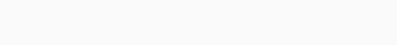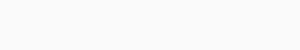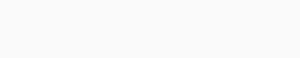# CPU Scheduling Algorithms – Preemptive or Non-Preemptive

CPU Scheduling Algorithms – Preemptive or Non-Preemptive :

A different processes to be assigned to the CPU Scheduling Algorithms based on particular algorithms. There are six popular process scheduling algorithms which we are going to discuss are as follows: First-Come, First-Served (FCFS) SchedulingShortest-Job-Next (SJN) SchedulingPriority SchedulingShortest Remaining TimeRound Robin(RR) SchedulingMultiple-Level Queues Scheduling. HRRN Scheduling.

## The Purpose of a CPU Scheduling Algorithms

• Maximum CPU utilization
• Fare allocation of CPU
• Maximum throughput
• Minimum turnaround time
• Minimum waiting time
• Minimum response time

## Scheduling Criteria

 Turnaround time This is the interval of time between the submission of a process and its com- pletion. Includes actual execution time plus time spent waiting for resources, including the processor. This is an appropriate measure for a batch job. Response time For an interactive process, this is the time from the submission of a request until the response begins to be received. Often a process can begin producing some output to the user while continuing to process the request. Thus, this is a better measure than turnaround time from the user’s point of view Deadlines When process completion deadlines can be specified, the scheduling discipline should subordinate other goals to that of maximizing the percentage of deadlines met. Predictability A given job should run in about the same amount of time and at about the same cost regardless of the load on the system. A wide variation in response time or turnaround time is distracting to users. It may signal a wide swing in system workloads or the need for system tuning to cure instabilities Throughput The scheduling policy should attempt to maximize the number of processes completed per unit of time. This is a measure of how much work is being performed. This clearly depends on the average length of a process but is also influenced by the scheduling policy, which may affect utilization. Processor utilization This is the percentage of time that the processor is busy. For an expensive shared system, this is a significant criterion. In single-user systems and in some other systems, such as real-time systems, this criterion is less important than some of the others. Fairness In the absence of guidance from the user or other system-supplied guidance, processes should be treated the same, and no process should suffer starvation. Enforcing priorities When processes are assigned priorities, the scheduling policy should favor higher-priority processes. Balancing resources The scheduling policy should keep the resources of the system busy. Processes that will underutilize stressed resources should be favored. This criterion also involves medium-term and long-term scheduling.

## Decision Mode

The decision mode specifies the instants in time at which the selection function is exercised. There are two general categories:

• Non preemptive: In this case, once a process is in the Running state, it continues to execute until
•  it terminates.
• it blocks itself to wait for I/O or to request some operating system service.
• Preemptive: The currently running process may be interrupted and moved to the Ready state by the operating system. The decision to preempt may be performed when a new process arrives; when an interrupt occurs that places a blocked process in the Ready state; or periodically, based on a clock interrupt.

## First-Come-First-Served (FCFS)

The simplest scheduling policy is first-come-first served (FCFS), also known as first-in-first-out (FIFO) or CPU Scheduling Algorithms a strict queuing scheme. As each process becomes ready, it joins the ready queue. When the currently running process ceases to execute, the process that has been in the ready queue the longest is selected for running.

• Simple
• Easy and useful and understand
• First come, first served

• This scheduling method is non preemptive, that is, the process will run until it finishes.
• Because of this nonpreemptive scheduling, short processes which are at the back of the queue have to   wait for the long process at the front to finish throughput is not efficient.
• It is used in a small system only where I/O efficiency is not very important

The Turn around time and the waiting time are calculated by using the following formula
.

• Turn Around Time = Completion Time – Arrival Time.
• Waiting Time = Turn Around Time – Burst Time.

### Example:

 AT BT CT 012 3 5 3 8 6 58 16 22

### Solutions:

 Process AT BT CT TAT=CT-AT WT=TAT-BT P0 P1P2P3 0 1 23 538 6 5 816 22 57 1419 5714 19

Gantt Chart show in the following:Average Wait Time: (0+4+6+13) / 4 = 5.75

Average Turn Around Time: (5+7+14+19)/4 = 30.75

## Shortest-Job-First (SJF) Scheduling

The SJF scheduler is exactly like FCFS except that instead of choosing the job at the front of the queue, it will always choose the shortest job (i.e. the job that takes the least time) available. We will use a sorted list to order the processes from longest to shortest.

• Associate with each process the length of its next CPU burst.
• Use these lengths to schedule the process with the shortest time.

#### There are two SJF scheduling algorithms:

• Non-Preemptive: Once CPU given to the process it cannot be preempted until completes its CPU burst. SJF is optimal – gives minimum average waiting time for
a given set of processes.
• Preemptive: If a new process arrives with CPU burst length less than remaining time of current executing process, preempt. This scheme is know as the Shortest-Remaining-Time-First (SRTF)

### Example:

#### Non-Preemptive SJF

 Process AT BT p1 p2p3p4 024 5 7414

### Solutions:

 Process AT BT CT TAT WT p1 p2p3p4 0245 7414 781216 76811 0277

Gantt Chart show in the following:Average Wait Time: (0+2+7+7) / 4 = 4

Average Turn Around Time: (7+6+8+11)/4 = 8

### SJF Preemptive Scheduling (Shortest Remaining Time)

Shortest remaining time (SRT) is the preemptive version of the SJF algorithm.
The processor is allocated to the job closest to completion but it can be preempted by a newer ready job with shorter time to completion.
It is often used in batch environments where short jobs need to give preference.

### Example:CT

p1=32

p2=6

p3=12

p4=5

Turn Around Time= CT-AT

p1= 32-0=32

p2=6-1=5

p3=12-2=10

p4=5-3=2

Wait Time

p1=32-21=11

p2=6-3=3

p3=12-6=6

p4=5-2=3

Average Wait Time: (11+3+6+3)/4=5.75

Average Turn Around Time: (32+5+10+2)/4 = 12.25

## Round Robin Scheduling Algorithms (RR)

•  The simplest such policy is round robin. A clock interrupt is generated at periodic intervals. When the interrupt occurs, the currently running process is placed in the ready queue, and the next ready job is selected on a FCFS basis.
• This technique is also known as time slicing, because each process is given a slice of time before being preempted.
• If the quantum is very short, then short processes will move through the system relatively quickly.
• There is processing overhead involved in handling the clock interrupt and performing the scheduling and dispatching function.
• Round robin is particularly effective in a general-purpose time-sharing system or transaction processing system. One drawback to round robin is its relative treatment of processor-bound and I/O-bound processes.Diagram of Round Robin Scheduling

#### CPU Scheduling Algorithms

• Every Thread / Process gets a chance to run.
• CPU is shared between all processes.
• Threads with the same priority are handled perfectly with Round Robin.

• Low Priority tasks may wait for more time if the many tasks are given high priority.
• High Priority tasks may not execute the full instruction given stipulated amount of time.

### Example:

 Process AT BT p1p2p3p4p5p6 012345 563154

### Solutions:

 process AT BT CT TAT WT p1p2p3p4p5p6 012345 563154 172311122421 1722992015 1216681511

Gantt Chart for Round Robin are as follows:Average Wait Time: 92/6=15.33

Average Turn Around Time: 68/6 = 11.33

## Priority Scheduling

• The SJF algorithm is a special case of the general priority scheduling algorithm.
• A priority is associated with each process, and the CPU is allocated to the process
with the highest priority. Equal-priority processes are scheduled in FCFS order.
• Priority scheduling can be either preemptive or nonpreemptive.
• When a process arrives at the ready queue, its priority is compared with the priority
of the currently running process.
• A preemptive priority scheduling algorithm will preempt the CPU if the priority of the newly arrived process is higher than the priority of the currently running process.
• A nonpreemptive priority scheduling algorithm will simply put the new process at the head of the ready queue.

• Simplicity.
• Reasonable support for priority.
• Suitable for applications with varying time and resource requirements.

• Indefinite blocking or starvation.
• A priority scheduling can leave some low priority waiting processes indefinitely for CPU.
• If the system eventually crashes then all unfinished low priority processes gets lost.

Example:

 Process BT AT Priority p1p2p3p4 21362 1032 2143

### Solutions:

 Process AT BT Priority CT TAT WT RT p1p2p3p4 1032 21362 2143 2433226 2332924 202322 102624

Gantt Chart for Priority Scheduling are as follows:Average waiting Time: (2+o+23+22)/4=11.75

Average Turn Around Time: (23+3+29+24)/4=19.75

## Highest Response Ratio Next (HRRN)

• Conventional HRRN algorithm schedules the tasks based on the response ratio which
provides the solution for starvation problem of scheduling algorithms.
• Response ratio for each task is based on estimated burst time and waiting time. Due to the absence of exact measure value of burst time, there may be chances of imprecise value.
• Some algorithms use fuzzy techniques to handle this impreciseness. These fuzzy heuristics dealt with impreciseness at some extent.
• Apart from this, these scheduling algorithms have also improved the performance of existing scheduling algorithms.

Consider the following ratio:

R=(w+s)/s

where

• R response ratio
• w time spent waiting for the processor
• s expected service time

If the process with this value is dispatched immediately, R is equal to the normalized
turnaround time. Note that the minimum value of R is 1.0, which occurs when a
process first enters the system.

### Example:

 Process AT BT p0p1p2p3p4 02468 35412

### Solutions:

 Process AT BT CT TAT WT p0p1p2p3p4 02468 35412 3813915 36937 01525

Gantt Chart for HRRN are as follows:• P1 is executed for 5 units. Meanwhile, all the processes get available. We have to calculate the Response Ratio for all the remaining jobs.

RR(P2)=((8-4)+4)/4=2

RR (P3)=(2+1)/1=3

RR (0+2)/2=1

Since, the Response ratio of P3 is higher hence P3 will be scheduled first.

• P3 is scheduled for 1 unit. The next available processes are P2 and P4. Let’s calculate their Response ratio.

RR (P2)=(5+2)/4=2.25

RR (P4)=(1+2)/2=1.5

The response ratio of P2 is higher hence P2 will be scheduled.

Average Wait Time: 13/5= 2.6

Average Turn Around Time: 28/5= 5.6

Reference

https://www.cl.cam.ac.uk/teaching/1617/OpSystems/pdf/05-Scheduling-Algorithms.pdf

https://www.javatpoint.com/os-scheduling-algorithms

https://en.wikipedia.org/wiki/Scheduling_(computing)

### You May Like

[table id=1 /]

error: Content is protected !!
Scroll to Top
Scroll to Top